Function Repository Resource:

# Immanant

Compute the immanant of a square matrix associated with an integer partition

Contributed by: Jan Mangaldan
 ResourceFunction["Immanant"][p,m] gives the immanant of the square matrix m associated with the integer partition p.

## Details

ResourceFunction["Immanant"] works with both numeric and symbolic matrices.
The immanant of an n×n matrix m associated with the integer partition p is given by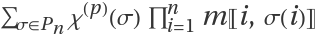, where χ(p)(σ) is the character of the symmetric group corresponding to p and the Pn are the permutations of n elements.
The immanant is a generalization of the determinant and the permanent of a square matrix.
If m has dimensions n×n, then p must be a weakly decreasing list of positive integers that sum to n.
The immanant is a polynomial of degree n in its entries.

## Examples

### Basic Examples (1)

Immanants of a 2×2 symbolic matrix:

 In:=Out=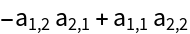In:=Out=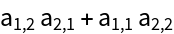### Scope (2)

All immanants of a 3×3 symbolic matrix:

 In:=Out=Use exact arithmetic to compute the immanant:

 In:=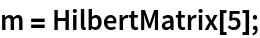In:=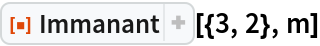Out=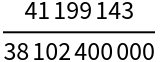Use machine arithmetic:

 In:=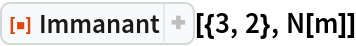Out=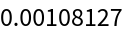Use 24-digit precision arithmetic:

 In:=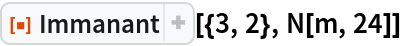Out=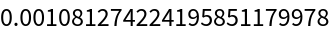### Applications (3)

Two graphs:

 In:=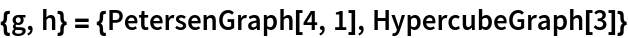Out=The immanantal polynomial of a graph is the immanant of id x-m, where m is the corresponding adjacency matrix and id is the identity matrix of appropriate size.

Prove that two graphs are isomorphic by showing that all their corresponding immanantal polynomials are identical:

 In:=In:=Out=This is consistent with the result of IsomorphicGraphQ:

 In:=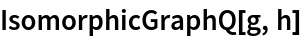Out=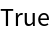### Properties and Relations (3)

The determinant is the immanant corresponding to the integer partition (1,1,…):

 In:=Out=The permanent is the immanant corresponding to the integer partition (n):

 In:=Out=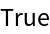Immanants are invariant under a symmetric permutation of rows and columns:

 In:=Out=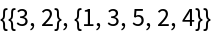In:=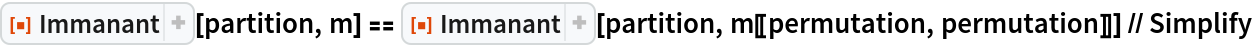Out=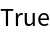### Possible Issues (2)

Immanant evaluates only if the integer partition p is a weakly decreasing list of positive integers:

 In:=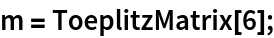In:=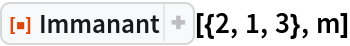Out=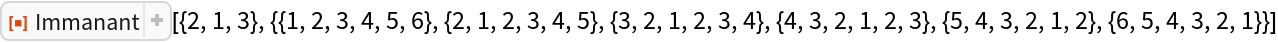In:=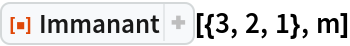Out=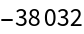In general, computing the immanant becomes slow even at modest dimension:

 In:=Out=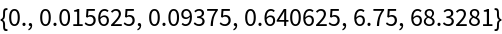In:=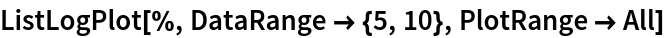Out=### Neat Examples (1)

Verify an identity for the immanant corresponding to the partition (3, 1, 1, …) according to Merris and Watkins (see citation in Source Metadata below):

 In:=Out=In:=Out=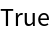## Version History

• 1.0.0 – 24 January 2022